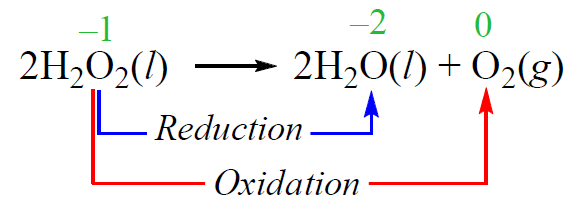## General Chemistry

Oxidation-reduction, or redox, reactions are electron transfer reactions. Because of electron transfers, the oxidation states of some atoms are changed in redox reactions.

• The atom that accepts an electron(s) is said to be reduced, and the one that gives the electron(s) is said to be oxidized.
• Oxidation is the process of losing an electron(s), and the reduction is the process of gaining an electron(s).

Historically, the word oxidation is referred to the combination of an element with oxygen to yield an oxide. And this is a good reference for classifying the process as an oxidation or reduction.

For example, in the following reaction, the changes in oxidation numbers are a result of electron transfers from Mg to O:• The oxidation number of Mg has increased – it has been oxidized.
• The oxidation number of oxygen has decreased – it has been reduced.

Oxygen is the oxidizing agent – it oxidizes Mg and thus, is reduced.

Mg is the reducing agent – it reduces oxygen and thus is oxidized. So, remember, in redox reactions:

• The oxidizing agent is being reduced.
• The reducing agent is being oxidized.

For example, identify the element that is oxidized and the element that is reduced in the following redox reaction:

2KMnO4 + 16HCl  → 2MnCl2 + 5Cl2 + 2KCl + 8H2O

Remember, not all the atoms undergo oxidation or reduction in a redox reaction. So, to find the species that undergo a redox transformation, look for atoms that are in different forms on both sides of the equation. For example, in the reaction, we see that chlorine is in a free form on the right side of the equation while on the left side, it is a chloride ion.  The manganese is also in a different environment, and it would be a good idea to compare its oxidation states.

The hydrogen and oxygen are always in their standard oxidation state unless they are in free forms. The exceptions of hydrogen are also when it is bound to a metal, while oxygen deviates from the standard -2 when connected to fluorine or to itself like in hydrogen peroxide. These exceptions are mentioned in the rules we discussed earlier.

So, the candidate molecules are KMnO4, MnCl2, HCl, and Cl2.There is quite a lot of terminology in redox reactions and it can get confusing. There are different ways of memorizing them.

For example, going with oil rig:

Oxidation is a loss (of electrons)

Reduction is a gain (of electrons)

I’d recommend going with oxygen. In most reactions, it is an oxidizing agent and goes from 0 to -2. So, you can go based on the fact that the oxidation number of oxidizing agent decreases. The most common oxidation reaction is probably the combustion reaction where O2 is the oxidizing agent.

# Disproportionation Reaction

In a disproportionation reaction, an element is simultaneously oxidized and reduced. For example, hydrogen peroxide decomposes to water and oxygen when exposed to light. While the oxidation state of hydrogen does not change, the oxygen undergoes oxidation and reduction:More examples of the oxidation state and redox reactions in this multiple-choice quiz:

Check Also